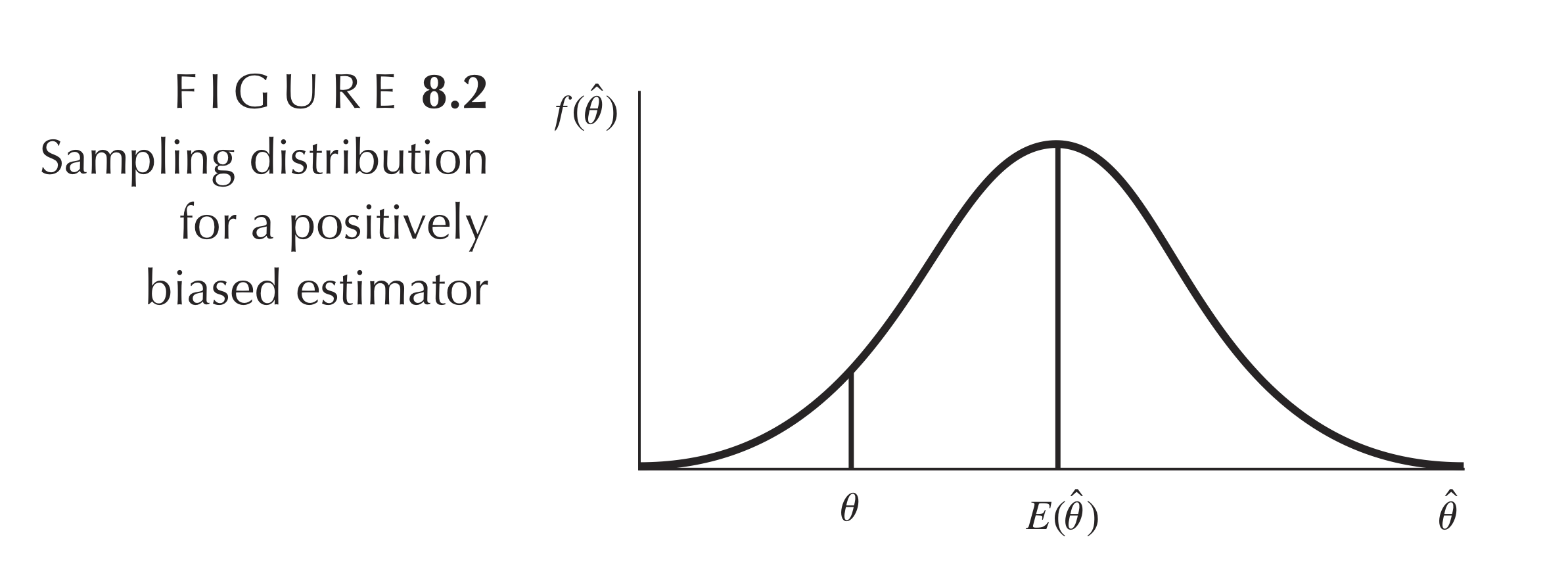# 最小二乘法的利与弊：高斯马尔科夫定理¶

《最小二乘法线性回归：矩阵视角》 一文里，我提到高斯马尔科夫定理证明了最小二乘法线性回归（OLS，下同）有一些特别好的性质，具体来说，当误差项均值为 0 时，OLS 得到的 $w$ 无偏（unbiased），如果各误差项方差相同，OLS 得到的 $w$ 是最佳无偏线性估计（BLUE, best linear unbiased estimator）。这篇文章里我会解释什么是无偏、什么是最佳无偏线性估计、如何证明 OLS 具有这些性质，并由此展开讨论 OLS 的局限。

## 如何评价 OLS？¶## 期望、协方差¶

$$w = \begin{bmatrix} w_0 \\ w_1 \\ w_2 \end{bmatrix} ,\quad \mathrm{E}(w) = \begin{bmatrix} \mathrm{E}(w_0) \\ \mathrm{E}(w_1) \\ \mathrm{E}(w_2) \end{bmatrix}=\begin{bmatrix} \bar{w_0} \\ \bar{w_1} \\ \bar{w_2} \end{bmatrix}$$

$$\begin{equation} \begin{split} \mathrm{Var}(w) &= \mathrm{E}\big[ [w - \mathrm{E}(w)][w - \mathrm{E}(w)]^T \big]\\ &= \mathrm{E}\Bigg[ \begin{pmatrix} w_0 - \bar{w_0} \\ w_1 - \bar{w_1} \\ w_2 - \bar{w_2} \end{pmatrix} \begin{pmatrix} w_0 - \bar{w_0} & w_1 - \bar{w_1} & w_2 - \bar{w_2} \end{pmatrix} \Bigg]\\ &=\begin{bmatrix} \mathrm{E}[(w_0 - \bar{w_0})(w_0 - \bar{w_0})] & \mathrm{E}[(w_0 - \bar{w_0})(w_1 - \bar{w_1})] & \mathrm{E}[(w_0 - \bar{w_0})(w_2 - \bar{w_2})] \\ \mathrm{E}[(w_1 - \bar{w_1})(w_0 - \bar{w_0})] & \mathrm{E}[(w_1 - \bar{w_1})(w_1 - \bar{w_1})] & \mathrm{E}[(w_1 - \bar{w_1})(w_2 - \bar{w_2})] \\ \mathrm{E}[(w_2 - \bar{w_2})(w_0 - \bar{w_0})] & \mathrm{E}[(w_2 - \bar{w_2})(w_1 - \bar{w_1})] & \mathrm{E}[(w_2 - \bar{w_2})(w_2 - \bar{w_2})] \end{bmatrix} \\ &= \begin{bmatrix} \mathrm{Var}(w_0) & \mathrm{Cov}(w_0 , w_1) & \mathrm{Cov}(w_0 , w_2) \\ \mathrm{Cov}(w_0 , w_1) & \mathrm{Var}(w_1) & \mathrm{Cov}(w_1 , w_2) \\ \mathrm{Cov}(w_0 , w_2) & \mathrm{Cov}(w_1 , w_2) & \mathrm{Var}(w_2) \\ \end{bmatrix} \end{split} \end{equation}$$

$$\begin{equation} \begin{split} \mathrm{E}(A+B) &= \mathrm{E}(A) +\mathrm{E}(B)\\ \mathrm{E}(A^2) &= \mathrm{E}(A)^2 + \mathrm{Var}(A) = \mu^2+\sigma^2\\ \mathrm{Cov}(A,B) &= \mathrm{E}(AB) - \mathrm{E}(A)\mathrm{E}(B)\\ \mathrm{Cov}(A,B) &= 0 \quad 当且仅当\ A,B \ 相互独立 \\ \end{split} \end{equation}$$

## $\hat{w}$ 的期望¶

$$\begin{equation} \begin{split} \mathrm{E}(\hat{w}) &= \mathrm{E}[(X^TX)^{-1}X^Ty] \\ &= \mathrm{E}[(X^TX)^{-1}X^T(Xw + \epsilon)] \\ &= \mathrm{E}[(X^TX)^{-1}X^TXw] + \mathrm{E}[(X^TX)^{-1}X^T\epsilon] \Longleftarrow (X^TX)^{-1}X^TX = I\\ &= \mathrm{E}[w] + \mathrm{E}[(X^TX)^{-1}X^T\epsilon] \end{split} \end{equation}$$

$$\begin{equation} \begin{split} E(\hat{w}) &= \mathrm{E}[w] + \mathrm{E}[(X^TX)^{-1}X^T\epsilon] \\ &= \mathrm{E}[w] + 0 \\ &= w \\ \end{split} \end{equation}$$

## $\hat{w}$ 的协方差矩阵¶

$$\begin{equation} \begin{split} \hat{w} &= (X^TX)^{-1}X^Ty \\ &= (X^TX)^{-1}X^T(Xw + \epsilon) \\ &= (X^TX)^{-1}X^TXw + (X^TX)^{-1}X^T\epsilon \\ &= w + (X^TX)^{-1}X^T\epsilon \end{split} \end{equation}$$

$$\begin{equation} \begin{split} \mathrm{Var}(\hat{w}) &= \mathrm{E}\big[ [\hat{w} - \mathrm{E}(\hat{w})][\hat{w} - \mathrm{E}(\hat{w})]^T \big]\\ &= \mathrm{E}\big[ (\hat{w} - w)(\hat{w} - w)^T \big] \end{split} \end{equation}$$

$$\begin{equation} \begin{split} \mathrm{Var}(\hat{w}) &= \mathrm{E}\big[ (\hat{w} - w)(\hat{w} - w)^T \big] \\ &= \mathrm{E}\big[ [w + (X^TX)^{-1}X^T\epsilon - w][w + (X^TX)^{-1}X^T\epsilon - w]^T \big] \\ &= \mathrm{E}\big[ [(X^TX)^{-1}X^T\epsilon][(X^TX)^{-1}X^T\epsilon]^T \big] \\ &= \mathrm{E}\big[ (X^TX)^{-1}X^T\epsilon\epsilon^T X(X^TX)^{-1} \big] \end{split} \end{equation}$$

$$\begin{equation} \begin{split} \mathrm{Var}(\epsilon) &= \mathrm{E}\big[ [\epsilon - \mathrm{E}(\epsilon)][\epsilon - \mathrm{E}(\epsilon)]^T \big]\\ &= \mathrm{E}(\epsilon\epsilon^T) \\ & = \begin{bmatrix} \mathrm{E}(\epsilon_1^2) & \mathrm{E}(\epsilon_1\epsilon_2) & \dots& \mathrm{E}(\epsilon_1\epsilon_i)\\ \mathrm{E}(\epsilon_2\epsilon_1) & \mathrm{E}(\epsilon_2^2) & \dots& \mathrm{E}(\epsilon_2\epsilon_i)\\ \vdots & \vdots & \ddots& \vdots\\ \mathrm{E}(\epsilon_i\epsilon_1) & \mathrm{E}(\epsilon_i\epsilon_2) & \dots& \mathrm{E}(\epsilon_i^2)\\ \end{bmatrix} \end{split} \end{equation}$$

$$\begin{equation} \begin{split} \mathrm{Var}(\epsilon) &= \mathrm{E}(\epsilon\epsilon^T)\\ & = \begin{bmatrix} \mathrm{E}(\epsilon_1^2) & \mathrm{E}(\epsilon_1\epsilon_2) & \dots& \mathrm{E}(\epsilon_1\epsilon_i)\\ \mathrm{E}(\epsilon_2\epsilon_1) & \mathrm{E}(\epsilon_2^2) & \dots& \mathrm{E}(\epsilon_2\epsilon_i)\\ \vdots & \vdots & \ddots& \vdots\\ \mathrm{E}(\epsilon_i\epsilon_1) & \mathrm{E}(\epsilon_i\epsilon_2) & \dots& \mathrm{E}(\epsilon_i^2)\\ \end{bmatrix}\\ &= \begin{bmatrix} \sigma^2 & 0 & \dots & 0\\ 0 & \sigma^2 & \dots& 0\\ \vdots & \vdots & \ddots& \vdots\\ 0 & \mathrm{E}(\epsilon_i\epsilon_2) & \dots& \sigma^2\\ \end{bmatrix}\\ &=\sigma^2I \end{split} \end{equation}$$

$$\begin{equation} \begin{split} \mathrm{Var}(\hat{w}) &= \mathrm{E}\big[ (X^TX)^{-1}X^T\epsilon\epsilon^T X(X^TX)^{-1} \big] \\ &= (X^TX)^{-1}X^T\mathrm{E}(\epsilon\epsilon^T) X(X^TX)^{-1} \\ &= (X^TX)^{-1}X^T\sigma^2 I X(X^TX)^{-1} \\ &= \sigma^2 (X^TX)^{-1}X^TI X(X^TX)^{-1} \\ &= \sigma^2 (X^TX)^{-1} \end{split} \end{equation}$$

## 高斯马尔科夫定理¶

$$\tilde{w} = My$$

$$\begin{equation} \begin{split} E(\tilde{w}) &= E(My) \\ &= E[M(Xw+\epsilon)] \\ &= E(MXw+M\epsilon) \\ &= E(MXw) \end{split} \end{equation}$$

$$\begin{equation} \begin{split} (X^TX)^{-1}X^T + C &= M \\ [(X^TX)^{-1}X^T + C]X &= MX \\ [(X^TX)^{-1}X^T + C]X &= I \\ (X^TX)^{-1}X^TX + CX &= I \\ CX &= 0 \end{split} \end{equation}$$

$$\begin{equation} \begin{split} \mathrm{Var}(\tilde{w}) &= \mathrm{E}\big[ [\tilde{w}-\mathrm{E}(\tilde{w})][\tilde{w}-\mathrm{E}(\tilde{w})]^T \big] \\ &= \mathrm{E}\big[ (\tilde{w}-w)(\tilde{w}-w)^T \big] \\ &= \mathrm{E}\big[ [M(Xw+\epsilon)-w][M(Xw+\epsilon)-w]^T \big] \\ &= \mathrm{E}\big[ (M\epsilon)(M\epsilon)^T \big] \\ &= \mathrm{E}(M\epsilon\epsilon^TM^T) \\ &= M\mathrm{E}(\epsilon\epsilon^T)M^T \\ &= \sigma^2MM^T \end{split} \end{equation}$$

$$\begin{equation} \begin{split} \mathrm{Var}(\tilde{w}) &= \sigma^2MM^T\\ &= \sigma^2[(X^TX)^{-1}X^T + C][(X^TX)^{-1}X^T + C]^T\\ &= \sigma^2\big[ (X^TX)^{-1}X^T[(X^TX)^{-1}X^T]^T + (X^TX)^{-1}X^TC^T + C[(X^TX)^{-1}X^T]^T + CC^T \big]\\ &= \sigma^2\big[ (X^TX)^{-1} + CC^T \big] \end{split} \end{equation}$$

$$\mathrm{Var}(\tilde{w}) - \mathrm{Var}(\hat{w}) = \sigma^2\big[ (X^TX)^{-1} + CC^T \big] - \sigma^2(X^TX)^{-1} = \sigma^2(CC^T)$$

## 最小二乘法的局限¶

$$\begin{equation} \begin{split} MSE &= \mathrm{E}[(\theta - \hat{\theta})^2]\\ &= \mathrm{E}\big[ [\theta - \mathrm{E}(\hat{\theta}) + \mathrm{E}(\hat{\theta}) - \hat{\theta}]^2 \big]\\ &= \mathrm{E}\big[ [\theta - \mathrm{E}(\hat{\theta})]^2 + [\hat{\theta}-\mathrm{E}(\hat{\theta})]^2 + 2[\theta - \mathrm{E}(\hat{\theta})][\mathrm{E}(\hat{\theta}) - \hat{\theta}] \big] \\ &= \mathrm{E}\big[ [\theta - \mathrm{E}(\hat{\theta})]^2\big] + \mathrm{E}\big[[\hat{\theta}-\mathrm{E}(\hat{\theta})]^2\big] + \mathrm{E}\big[2[\theta - \mathrm{E}(\hat{\theta})][\mathrm{E}(\hat{\theta}) - \hat{\theta}] \big] \\ \end{split} \end{equation}$$

$$\begin{equation} \begin{split} MSE &= \mathrm{E}[(\theta - \hat{\theta})^T (\theta - \hat{\theta})]\\ &= \mathrm{E}\big[ [\theta - \mathrm{E}(\hat{\theta})]^2\big] + \mathrm{E}\big[[\hat{\theta}-\mathrm{E}(\hat{\theta})]^2\big] + \mathrm{E}\big[2[\theta - \mathrm{E}(\hat{\theta})][\mathrm{E}(\hat{\theta}) - \hat{\theta}] \big] \\ &= \mathrm{Bias}^2(\hat{\theta}) + \mathrm{Var}(\hat{\theta})\\ \end{split} \end{equation}$$

## 修改与勘误¶

• 和我的计量经济学老师 Ioannis Karavias 讨论了 p 值重不重要，得出的结论是，重要。因为目前机器学习尚不能检验对于理论（假设）正不正确，只能得到预测值，可解释性需要打问号。
• 最后一部分证明有误，因为矩阵不能比较大小，需要一些比较 tricky 的方法，不想把证明搞复杂，所以改用半正定矩阵的性质解答。
• MSE 分解为偏差方差的公式中的 $w$ 应该为标量，为了与上面的证明区分，改用为标量 $\theta$。矩阵形式 MSE 分解应该存在，但对结论影响不大，暂时搁置，